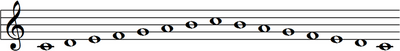# Interval (music) facts for kids

Kids Encyclopedia Facts

An interval in music means the distance between two notes (whether these notes are played together or separately).

Two notes on a keyboard which are next to one another (counting both white and black notes) are a “semitone” apart. A “tone” is two semitones (see semitone). However, an “intervale” depends on how the notes are written (what they are called) e.g. whether the black note between D and E is written as D sharp or E flat (which are two different names for the same note).Please note that in all of the examples below, C is the lower note of the pair, and the other note is the next note of that name above it. So, the interval between a C and a B is only a major 7th if it is between a C and next B above it.

## Major and perfect intervals in the major scale

In a major scale there are eight notes e.g. a C major scale (see above) is: C D E F G A B C and down again.

• The interval between C and D is a major 2nd (major second).
• The interval between C and E is a major 3rd (major third)
• The interval between C and F is a perfect 4th (perfect fourth)
• The interval between C and G is a perfect 5th (perfect fifth)
• The interval between C and A is a major 6th (major sixth)
• The interval between C and B is a major 7th (major seventh)
• The interval between C and C is a perfect 8th (perfect octave)

## Minor intervals

If a major interval is made smaller by a semitone it becomes minor:

• The interval between C and D flat is a minor 2nd
• The interval between C and E flat is a minor 3rd
• The interval between C and A flat is a minor 6th
• The interval between C and B flat is a minor 7th

## Diminished intervals

If a perfect interval becomes smaller by a semitone it becomes “diminished”

• The interval between C and F flat is a diminished 4th
• The interval between C and G flat is a diminished 5th

If a major interval becomes smaller by two semitones (i.e. a tone) it becomes “diminished”

• The interval between C and D double flat is a diminished 2nd
• The interval between C and E double flat is a diminished 3rd
• The interval between C and A double flat is a diminished 6th
• The interval between C and B double flat is a diminished 7th

## Augmented intervals

If a major interval is made bigger by a semitone it becomes “augmented”

• The interval between C and D sharp is an augmented 2nd
• The interval between C and E sharp is an augmented 3rd
• The interval between C and A sharp is an augmented 6th
• The interval between C and B sharp is an augmented 7th

Similarly, if a perfect interval becomes bigger by a semitone it becomes “augmented”

• The interval between C and F sharp is an augmented 4th
• The interval between C and G sharp is an augmented 5th

Examples:

• C to D sharp is an augmented 2nd (N.B. but if the D sharp is called E flat it is a minor 3rd)
• C to G flat is a diminished 5th
• C to B double flat (the same note as A) is a diminished 7th.

It is important to realize that, in order to work out what an interval is, the lower note should be treated as the first note of the scale.

Examples:

• C sharp to A sharp is a major 6th
• C sharp to A is a minor 6th

## Inverted intervals

Intervals can be “inverted” (turned upside down). For example: instead of going from a C up to an A (major 6th) we can go from C down to an A (minor 3rd).

• A major 6th is the inversion of a minor 3rd.
• All major intervals, when inverted, become minor intervals.
• All augmented intervals, when inverted, become diminished.
• All perfect intervals, when inverted, are still perfect (this is why they are called “perfect”).
• All intervals, when inverted, add up to 9 (there are 8 notes in a scale. One note is obviously being counted twice).

Compound intervals are intervals bigger than an octave e.g. C to D an octave and one more note above it is a major 9th.

Big intervals are called “wide” intervals. The opposite is a “narrow” interval.

## Images for kidsInterval (music) Facts for Kids. Kiddle Encyclopedia.